GATE  >  Instrumentation Engineering - IN 2012 GATE Paper (Practice Test)

# Instrumentation Engineering - IN 2012 GATE Paper (Practice Test) - GATE

Test Description

## 65 Questions MCQ Test GATE Past Year Papers for Practice (All Branches) - Instrumentation Engineering - IN 2012 GATE Paper (Practice Test)

Instrumentation Engineering - IN 2012 GATE Paper (Practice Test) for GATE 2023 is part of GATE Past Year Papers for Practice (All Branches) preparation. The Instrumentation Engineering - IN 2012 GATE Paper (Practice Test) questions and answers have been prepared according to the GATE exam syllabus.The Instrumentation Engineering - IN 2012 GATE Paper (Practice Test) MCQs are made for GATE 2023 Exam. Find important definitions, questions, notes, meanings, examples, exercises, MCQs and online tests for Instrumentation Engineering - IN 2012 GATE Paper (Practice Test) below.
Solutions of Instrumentation Engineering - IN 2012 GATE Paper (Practice Test) questions in English are available as part of our GATE Past Year Papers for Practice (All Branches) for GATE & Instrumentation Engineering - IN 2012 GATE Paper (Practice Test) solutions in Hindi for GATE Past Year Papers for Practice (All Branches) course. Download more important topics, notes, lectures and mock test series for GATE Exam by signing up for free. Attempt Instrumentation Engineering - IN 2012 GATE Paper (Practice Test) | 65 questions in 180 minutes | Mock test for GATE preparation | Free important questions MCQ to study GATE Past Year Papers for Practice (All Branches) for GATE Exam | Download free PDF with solutions
 1 Crore+ students have signed up on EduRev. Have you?
Instrumentation Engineering - IN 2012 GATE Paper (Practice Test) - Question 1

### Q. 1 – Q. 5 carry one mark each. Choose the most appropriate alternative from the options given below to complete the following sentence: If the tired soldier wanted to lie down, he ___ the mattress out on the balcony.

Instrumentation Engineering - IN 2012 GATE Paper (Practice Test) - Question 2

### If (1.001)1259 = 3.52 and (1.001)2062 = 7.85, then (1.001)3321 =

Instrumentation Engineering - IN 2012 GATE Paper (Practice Test) - Question 3

### One of the parts (A, B, C, D) in the sentence given below contains an ERROR. Which one of the following is INCORRECT? I requested that he should be given the driving test today instead of tomorrow.

Instrumentation Engineering - IN 2012 GATE Paper (Practice Test) - Question 4

Which one of the following options is the closest in meaning to the word given below?

Latitude

Instrumentation Engineering - IN 2012 GATE Paper (Practice Test) - Question 5

Choose the most appropriate word from the options given below to complete the following sentence:

Given the seriousness of the situation that he had to face, his ___ was impressive.

Instrumentation Engineering - IN 2012 GATE Paper (Practice Test) - Question 6

Q. 6 - Q. 10 carry two marks each.

Q. Raju has 14 currency notes in his pocket consisting of only Rs. 20 notes and Rs. 10 notes. The total
money value of the notes is Rs. 230. The number of Rs. 10 notes that Raju has is

Instrumentation Engineering - IN 2012 GATE Paper (Practice Test) - Question 7

One of the legacies of the Roman legions was discipline. In the legions, military law prevailed and discipline was brutal. Discipline on the battlefield kept units obedient, intact and fighting, even when the odds and conditions were against them.

Which one of the following statements best sums up the meaning of the above passage?

Instrumentation Engineering - IN 2012 GATE Paper (Practice Test) - Question 8

A and B are friends. They decide to meet between 1 PM and 2 PM on a given day. There is a condition that whoever arrives first will not wait for the other for more than 15 minutes. The probability that they will meet on that day is

Instrumentation Engineering - IN 2012 GATE Paper (Practice Test) - Question 9

The data given in the following table summarizes the monthly budget of an average household.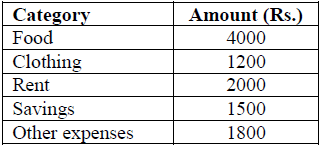The approximate percentage of the monthly budget NOT spent on savings is

Instrumentation Engineering - IN 2012 GATE Paper (Practice Test) - Question 10

There are eight bags of rice looking alike, seven of which have equal weight and one is slightly heavier. The weighing balance is of unlimited capacity. Using this balance, the minimum number of weighings required to identify the heavier bag is

Instrumentation Engineering - IN 2012 GATE Paper (Practice Test) - Question 11

Q. 1 – Q. 25 carry one mark each.

Q. If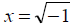, then the value of xx is

Instrumentation Engineering - IN 2012 GATE Paper (Practice Test) - Question 12

With initial condition x(1) = 0.5 , the solution of the differential equation,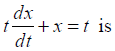Instrumentation Engineering - IN 2012 GATE Paper (Practice Test) - Question 13

Two independent random variables X and Y are uniformly distributed in the interval [–1, 1]. The probability that max[X, Y] is less than 1/2 is

Instrumentation Engineering - IN 2012 GATE Paper (Practice Test) - Question 14

The unilateral Laplace transform of f (t) is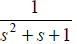. The unilateral Laplace transform of  tf(t) is

Instrumentation Engineering - IN 2012 GATE Paper (Practice Test) - Question 15

Given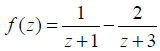. If C is a counterclockwise path in the z-plane such that |z +1| =1, the value of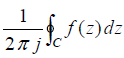is

Instrumentation Engineering - IN 2012 GATE Paper (Practice Test) - Question 16

The average power delivered to an impedance (4 - j3)? by a current 5cos(100πt +100) A is

Instrumentation Engineering - IN 2012 GATE Paper (Practice Test) - Question 17

In the circuit shown below, the current through the inductor is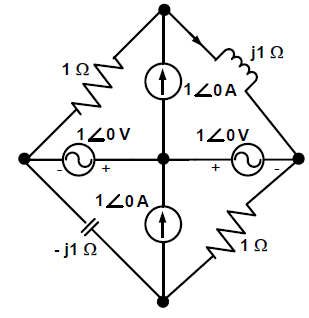Instrumentation Engineering - IN 2012 GATE Paper (Practice Test) - Question 18

I n the following figure, C1 and C2 are ideal capacitors. C1 has been charged to 12 V before the ideal
switch S is closed at t = 0. The current i(t) for all t is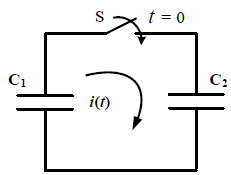Instrumentation Engineering - IN 2012 GATE Paper (Practice Test) - Question 19

T he impedance looking into nodes 1 and 2 in the given circuit is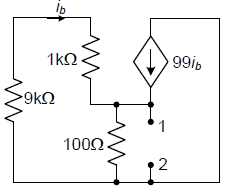Instrumentation Engineering - IN 2012 GATE Paper (Practice Test) - Question 20

T he i-v characteristics of the diode in the circuit given below are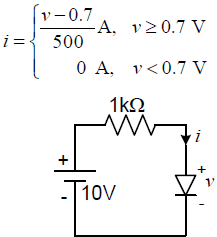The current in the circuit is

Instrumentation Engineering - IN 2012 GATE Paper (Practice Test) - Question 21

A system with transfer function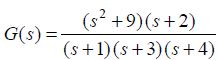is excited by sin(ω t) . The steady-state output of the system is zero at

Instrumentation Engineering - IN 2012 GATE Paper (Practice Test) - Question 22

The output Y of a 2-bit comparator is logic 1 whenever the 2-bit input A is greater than the 2-bit input B. The number of combinations for which the output is logic 1, is

Instrumentation Engineering - IN 2012 GATE Paper (Practice Test) - Question 23

In the sum of products function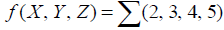, the prime implicants are

Instrumentation Engineering - IN 2012 GATE Paper (Practice Test) - Question 24

Consider the given circuit.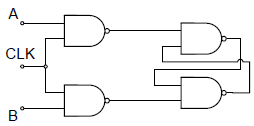In this circuit, the race around

Instrumentation Engineering - IN 2012 GATE Paper (Practice Test) - Question 25

If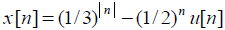, then the region of convergence (ROC) of its Z-transform in the Z-plane will be

Instrumentation Engineering - IN 2012 GATE Paper (Practice Test) - Question 26

A capacitive motion transducer circuit is shown. The gap d between the parallel plates of the capacitor is varied as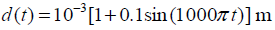. If the value of the capacitance is 2pF at
t = 0 ms, the output voltage Vo at t = 2 ms is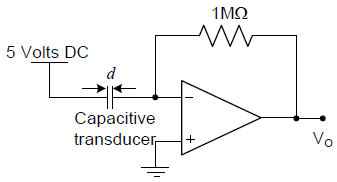Instrumentation Engineering - IN 2012 GATE Paper (Practice Test) - Question 27

A psychrometric chart is used to determine

Instrumentation Engineering - IN 2012 GATE Paper (Practice Test) - Question 28

A strain gauge is attached on a cantilever beam as shown. If the base of the cantilever vibrates according to the equation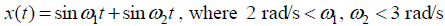, then the output of the strain gauge is proportional to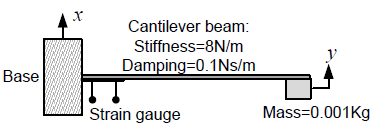Instrumentation Engineering - IN 2012 GATE Paper (Practice Test) - Question 29

T he transfer function of a Zero-Order-Hold system with sampling interval T is

Instrumentation Engineering - IN 2012 GATE Paper (Practice Test) - Question 30

A n LED emitting at 1 μm with a spectral width of 50 nm is used in a Michelson interferometer. To
obtain a sustained interference, the maximum optical path difference between the two arms of the
interferometer is

Instrumentation Engineering - IN 2012 GATE Paper (Practice Test) - Question 31

L ight of wavelength 630 nm in vacuum, falling normally on a biological specimen of thickness 10 μm, splits into two beams that are polarized at right angles. The refractive index of the tissue for the two polarizations are 1.32 and 1.333. When the two beams emerge, they are out of phase by

Instrumentation Engineering - IN 2012 GATE Paper (Practice Test) - Question 32

The responsivity of the PIN photodiode shown is 0.9 A/W. To obtain Vout of –1 V for an incident optical power of 1 mW, the value of R to be used is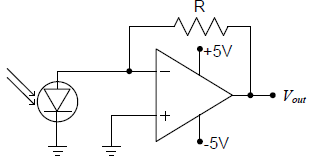Instrumentation Engineering - IN 2012 GATE Paper (Practice Test) - Question 33

A periodic voltage waveform observed on an oscilloscope across a load is shown. A permanent magnet moving coil (PMMC) meter connected across the same load reads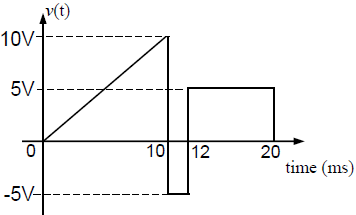Instrumentation Engineering - IN 2012 GATE Paper (Practice Test) - Question 34

For the circuit shown in the figure, the voltage and current expressions are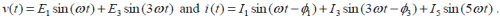The average power measured by the Wattmeter is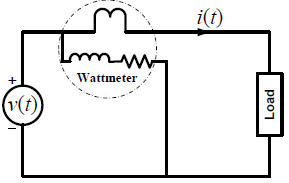Instrumentation Engineering - IN 2012 GATE Paper (Practice Test) - Question 35

The bridge method commonly used for finding mutual inductance is

Instrumentation Engineering - IN 2012 GATE Paper (Practice Test) - Question 36

Q. 36 - Q 65 carry two marks each:

A fair coin is tossed till a head appears for the first time. The probability that the number of required tosses is odd, is

Instrumentation Engineering - IN 2012 GATE Paper (Practice Test) - Question 37

Given that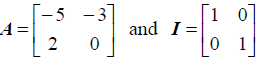the value of A3 is

Instrumentation Engineering - IN 2012 GATE Paper (Practice Test) - Question 38

The direction of vector A is radially outward from the origin, with |A| = krn where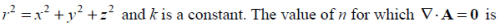Instrumentation Engineering - IN 2012 GATE Paper (Practice Test) - Question 39

The maximum value of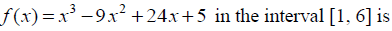Instrumentation Engineering - IN 2012 GATE Paper (Practice Test) - Question 40

Consider the differential equation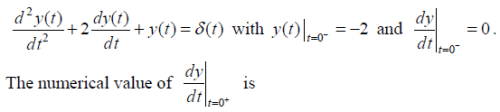Instrumentation Engineering - IN 2012 GATE Paper (Practice Test) - Question 41

If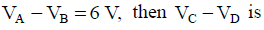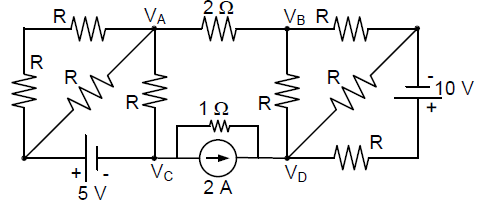Instrumentation Engineering - IN 2012 GATE Paper (Practice Test) - Question 42

A ssuming both the voltage sources are in phase, the value of R for which maximum power is transferred from circuit A to circuit B is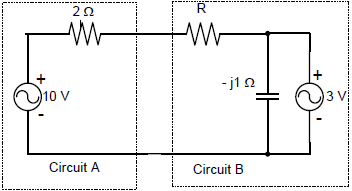Instrumentation Engineering - IN 2012 GATE Paper (Practice Test) - Question 43

The voltage gain Av of the circuit shown below is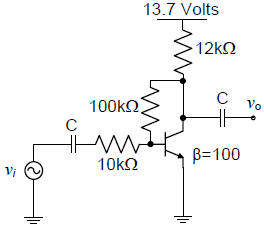Instrumentation Engineering - IN 2012 GATE Paper (Practice Test) - Question 44

The state variable description of an LTI system is given by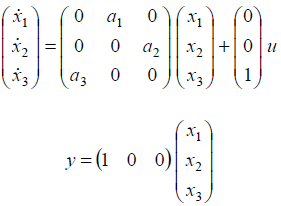where y is the output and u is the input. The system is controllable for

Instrumentation Engineering - IN 2012 GATE Paper (Practice Test) - Question 45

The state transition diagram for the logic circuit shown is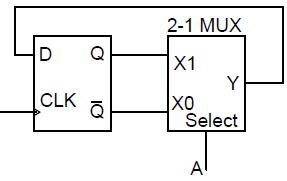Instrumentation Engineering - IN 2012 GATE Paper (Practice Test) - Question 46

The Fourier transform of a signal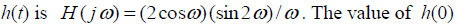is

Instrumentation Engineering - IN 2012 GATE Paper (Practice Test) - Question 47

L et y[n] denote the convolution of h[n] and g[n], where h[n] = (1/2)n u[n] and g[n] is a causal sequence. If y = 1 and y = 1/2, then g equals

Instrumentation Engineering - IN 2012 GATE Paper (Practice Test) - Question 48

The feedback system shown below oscillates at 2 rad/s when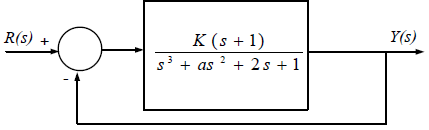Instrumentation Engineering - IN 2012 GATE Paper (Practice Test) - Question 49

The circuit shown is a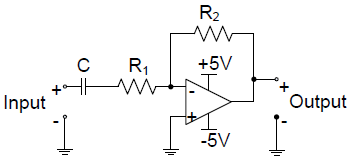Instrumentation Engineering - IN 2012 GATE Paper (Practice Test) - Question 50

The input x(t) and output y(t) of a system are related as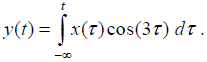The system is

Instrumentation Engineering - IN 2012 GATE Paper (Practice Test) - Question 51

A double convex lens is used to couple a laser beam of diameter 5 mm into an optical fiber with a numerical aperture of 0.5. The minimum focal length of the lens that should be used in order to focus the entire beam into the fiber is

Instrumentation Engineering - IN 2012 GATE Paper (Practice Test) - Question 52

A n analog voltmeter uses external multiplier settings. With a multiplier setting of 20 kilo-ohms it reads
440 V and with a multiplier setting of 80 kilo-ohms it reads 352 V. For a multiplier setting of 40 kilo-ohms the

Instrumentation Engineering - IN 2012 GATE Paper (Practice Test) - Question 53

The open loop transfer function of a unity negative feedback control system is given by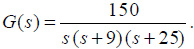.The gain margin of the system is

Instrumentation Engineering - IN 2012 GATE Paper (Practice Test) - Question 54

A dynamometer arm makes contact with the piezoelectric load cell as shown. The g-constant of the piezoelectric material is 50 x 10-3Vm/N and the surface area of the load cell is 4 cm2. If a torque τ =20 Nm is applied to the dynamometer, the output voltage Vo of the load cell is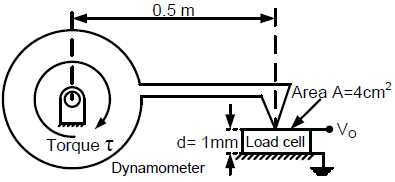Instrumentation Engineering - IN 2012 GATE Paper (Practice Test) - Question 55

Water (density: 1000 kgm–3) stored in a cylindrical drum of diameter 1 m is emptied through a horizontal pipe of diameter 0.05 m. A pitot-static tube is placed inside the pipe facing the flow. At the time when the difference between the stagnation and static pressures measured by the pitotstatic tube is 10 kPa, the rate of reduction in water level in the drum is,

Instrumentation Engineering - IN 2012 GATE Paper (Practice Test) - Question 56

A U-tube manometer of tube diameter D is filled with a liquid of zero viscosity. If the volume of the liquid filled is V, the natural frequency of oscillations in the liquid level about its mean position, due to small perturbations, is

Instrumentation Engineering - IN 2012 GATE Paper (Practice Test) - Question 57

The open loop transfer function of a unity gain negative feedback control system is given by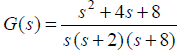. The angle θ , at which the root locus approaches the zeros of the system,
satisfies

Instrumentation Engineering - IN 2012 GATE Paper (Practice Test) - Question 58

Common Data Questions

Common Data for Questions 58 and 59:

With 10 V dc connected at port A in the linear nonreciprocal two-port network shown below, the following
were observed:

(i) 1 ohm connected at port B draws a current of 3 A

(ii) 2.5 ohm connected at port B draws a current of 2 A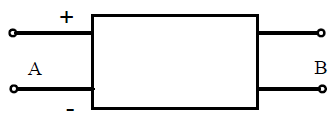Q. With 10 V dc connected at port A, the current drawn by 7 ohm connected at port B is

Instrumentation Engineering - IN 2012 GATE Paper (Practice Test) - Question 59

Common Data Questions

Common Data for Questions 58 and 59:

With 10 V dc connected at port A in the linear nonreciprocal two-port network shown below, the following
were observed:

(i) 1 ohm connected at port B draws a current of 3 A

(ii) 2.5 ohm connected at port B draws a current of 2 AQ. For the same network, with 6 V dc connected at port A, 1 ohm connected at port B draws 7/3 A.
If 8 V dc is connected to port A, the open circuit voltage at port B is

Instrumentation Engineering - IN 2012 GATE Paper (Practice Test) - Question 60

Common Data for Questions 60 and 61:

The deflection profile y (x) of a cantilever beam due to application of a point force F (in Newton), as a
function of distance x from its base, is given by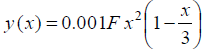. The angular deformation θ at the end of the cantilever is measured by reflecting a laser beam off a mirror M as shown in the figure.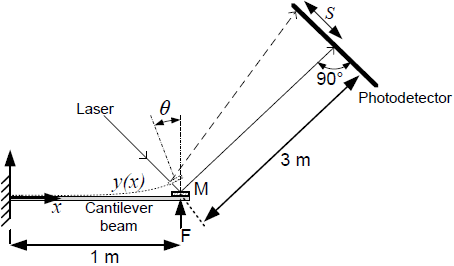Q. The translation S of the spot of laser light on the photodetector when a force of F =1 N is applied
to the cantilever is

Instrumentation Engineering - IN 2012 GATE Paper (Practice Test) - Question 61

Common Data for Questions 60 and 61:

The deflection profile y (x) of a cantilever beam due to application of a point force F (in Newton), as a
function of distance x from its base, is given by. The angular deformation θ at the end of the cantilever is measured by reflecting a laser beam off a mirror M as shown in the figure.If linear variable differential transformers (LVDTs) are mounted at x = 1/2 m and x = 1/4 m on the
cantilever to measure the effect of time varying forces, the ratio of their outputs is

Instrumentation Engineering - IN 2012 GATE Paper (Practice Test) - Question 62

The transfer function of a compensator is given as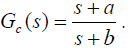Q. Gc(s) is a lead compensator if

Instrumentation Engineering - IN 2012 GATE Paper (Practice Test) - Question 63

The transfer function of a compensator is given asQ. The phase of the above lead compensator is maximum at

Instrumentation Engineering - IN 2012 GATE Paper (Practice Test) - Question 64

In the circuit shown, the three voltmeter readings are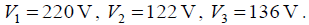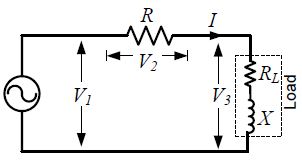Q. The power factor of the load is

Instrumentation Engineering - IN 2012 GATE Paper (Practice Test) - Question 65

In the circuit shown, the three voltmeter readings areQ. If RL = 5 ohm , the approximate power consumption in the load is

## GATE Past Year Papers for Practice (All Branches)

407 docs|127 tests
Information about Instrumentation Engineering - IN 2012 GATE Paper (Practice Test) Page
In this test you can find the Exam questions for Instrumentation Engineering - IN 2012 GATE Paper (Practice Test) solved & explained in the simplest way possible. Besides giving Questions and answers for Instrumentation Engineering - IN 2012 GATE Paper (Practice Test), EduRev gives you an ample number of Online tests for practice

## GATE Past Year Papers for Practice (All Branches)

407 docs|127 tests